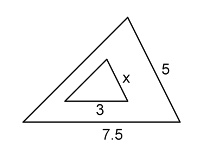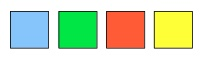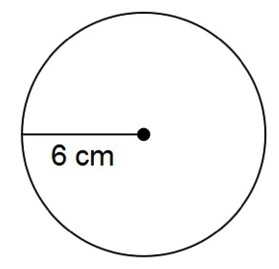Start Now with 100 Free Questions

# WIAT-4 (WIAT-IV) Achievement Testing – Mathematics

## What are the WIAT-4 (WIAT-IV) Mathematics Subtests?

The Mathematics Subtests on the WIAT-4 (WIAT-IV) are designed to measure a student’s mathematics proficiency, as well as their fluency or how quickly and accurately they can solve simple math facts. They assess a student’s math skills in a variety of areas, including basic computation, math fluency, math reasoning, and math problem-solving. The test consists of both oral and written questions, and it assesses a student’s ability to apply mathematical concepts to real-world situations.

The WIAT-4 Mathematics Subtest can provide useful information about a student’s math abilities, which can be used to identify areas of strength and weakness, inform educational programming and intervention strategies, and provide important information for educational planning. The subtests for the WIAT-4 (WIAT-IV) have not changed and are the same as the WIAT-III.

WIAT-4 (WIAT-IV) Mathematic subtests consist of numerical operations, math problem solving, and math fluency.  Below are some of the sample questions.

### Numerical Operations

This section measures the student’s math calculation skills which include solving written calculation problems and solving basic operations. Initially, the student will answer oral questions about number concepts and counting. Later, they will write answers to printed math problems ranging from basic operations to geometry and algebra.

Sample Question #1: Solve the following:

1  +  2  =  _____

Sample Question #2: Solve the following:

8  –  3  =  _____

Sample Question #3: Six children are playing football and 10 children are playing soccer. What is the ratio of the number of students who are playing football to those who are playing soccer?

Answer:  6:10; 6 children playing football to 10 children playing soccer is expressed as the ratio 6:10.

Sample Question #4:  The triangles in the figure below are similar.  What is the value of x?Answer:  2; 3 is to 7.5 as x is to 5; 3:7.5 = x:5, 3/7.5 = x/5, 15 = 7.5x, x =2

### Math – Problem Solving

This section assesses a student’s problem-solving skills, from reasoning, basic concepts to everyday mathematics applications, including geometry and algebra. Initially, students may be asked to point to an answer or respond orally. This is followed by problem solving questions that require the application of math principles to determine the answers. If needed, students can use empty spaces on the test sheet to work out the problems.

Sample Question #1:  Demi went to visit Sam in the hospital.  When she got to his room, 5 people were there.  3 left.  How many visitors were there while Demi was there?

Answer:  3; 5 – 3 = 2 (including Demi)

Sample Question #2:  If the pattern shown were to repeat itself, what color would the 20th square be?Sample Question #3: Which of the following expressions is 5 times smaller than the sum of 4 and 10?

1.  4 + 10/5
2.  4 + (10/5)
3.  (4 + 10) x 5
4.  (4 + 10)/5

Answer:  4.  (4 + 10)/5; If something is “5 times smaller than” then it is divided by 5.

Sample Question #4:  What is the diameter of the circle below?1.  6 cm
2.  12 cm
3.  6π cm
4.  12π cm

Answer:  2. 12cm; The diameter of a circle is a straight line that passes through the center of the circle and whose endpoints lie on the circumference of the circle. 6 cm is the given radius which is half the diameter. Therefore, the diameter is 6 x 2 = 12 cm.

### Math Fluency

This section measures math fact accuracy under timed conditions. The problems are presented in a vertical format.

In this 3-part math fluency test, there will be a list of math problems that consist of addition, subtraction, and multiplication.  The student has 60 seconds to answer as many addition problems as they can. Then they have 60 seconds to answer as many subtraction problems as they can.  Then they have 60 seconds to answer as many multiplication problems as they can.  Students are scored based on how many of each type of problem they complete accurately within 60 seconds.